# Quiz 9: The Impact of Variability on Process Performance: Throughput Losses

Business

In order to compute the probability of m resources being utilized and a newly arrived unit is rejected, the Erlang Loss Formula table has to be used. a. The average arrival rate is 55 units per hour. So, the average interarrival time,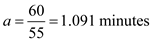The average processing time,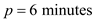The number of servers, m = 7 Look up the Erlang Loss Formula table for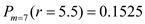So, the probability that all the seven servers are busy is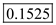b. Compute the flow rate, R using the following formula:So, the flow rate i.e. the customers served per hour is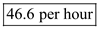c. Compute the customer lost customer per hour by subtracting the flow rate from the average arrival rate. So,So, customers lost per hour is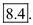Consider the following information: Number of guards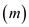= 5 Calls per hour = 12 calls Therefore, inter-arrival time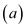= 60/12 = 5 minutes Activity time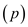= 90 minutes a. Determine the fraction of time the incoming alarms are directed to the police as shown below: Initially determine the ratio of activity time to inter-arrival time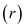using given formula: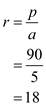Now, use the Erlang loss table to find the probability that that all five servers are utilized. For the table it is found that for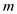= 5 and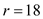the probability that all servers are busy is,Hence, the fraction of time the incoming alarms are directed to the police is 0.7402 or 74.02%. b. Determine the total amount the home security has to pay the local police every month as shown below: Determine the lost customer using given formula:The local police charges \$5000 for every call. Therefore, the total amount that home security pays every month to local police is calculated as shown below:Hence, the home security has to pay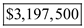to the local police.

The given information is shown below: Number of copies = 9 Arrival rate, A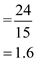Average Rental duration, P = 36 hours (a) Calculate the likelihood that a customer going to the video store would find the movie available as shown below :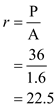Use "Erlang Loss" Table to find the probability with all utilized servers Probability of r (22.5) = 62.44% Thus, people going to video store would find the movie available with the probability of (100-62.44) = 37.56% (b) Calculate the revenue of the store per day as shown below: Flow rate = 0.235 customers per hour. The Revenue of the store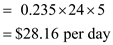Thus, the Revenue of the store per day is \$28.16 (c) The loss rate is 0.39 customers per hour and each of them get \$1. Calculate the loss for the day as shown below: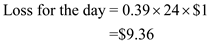Thus, loss for the day is \$9.36 (d) Number of copies = 9 Arrival rate, AAverage Rental duration, P = 36 hours Calculate the revenue of the store per day as shown below : Flow rate = 0.259 customers per hour. The Revenue of the store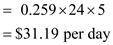Thus, the Revenue of the store per day is \$31.19 Thus, the Increased profit is (31.19 - 28.16) = \$3.62